# Electronics and Communication Engineering - Exam Questions Papers

1.
A lossless 50 Ω transmission line is terminated 30 + j25 Ω, then voltage reflection coefficient and current reflection coefficient are
062 ∠82.88, - 0.62 ∠82.88
10.36 ∠11.296, - 10.36 ∠111.296
0.23 ∠49.1, - 0.23 ∠ + 49.1
- 10.36 ∠111.296, 10.36 ∠111.296
Explanation:

Voltage reflection coefficient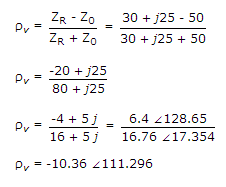Current reflection coefficient : ri = - rv = + 10.36 ∠111.296.

2.
Find value of i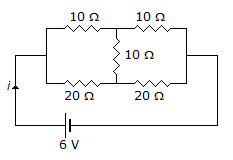1A
0A
0.45 A
None of these
Explanation:

The given circuit can be compared to a Wheatstone's Bridge.

This bridge is balanced.

Req = (10 + 10) || (20 + 20) = 20 || 40 = 13.33 Ω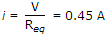.

3.
The circuit shown in the figure has 4 boxes each described by inputs P, Q, R and outputs Y, Z with
Y = P ⊕ Q ⊕ R, Z = RQ + P R + Q P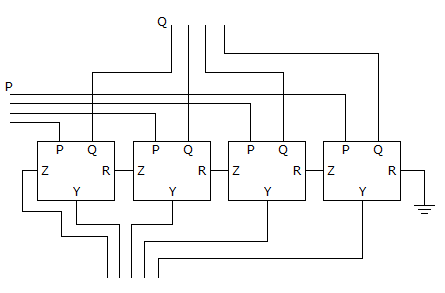The circuit is a
4 bit adder giving P + Q
4 bit subtractor giving P - Q
4 bit subtractor giving Q - P
4 bit adder giving P + Q + R
Explanation:

Let P = 1101 Q = 1101

Yn = Pn ⊕ Qn ⊕ Rn

Z = Rn Qn + Pn Rn + Qn Pn

Constructing truth table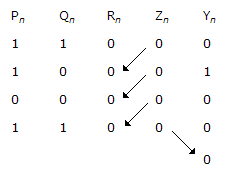So that, Rn + 1 = Zn 1 ≥ n ≥ 3

Z4 = R5(MSB)

Hence, output is 00010 which show that it is a 4 bit subtractor giving P - Q.

4.
Find the electric charge required on the earth and moon to balance their gravitational attraction if the charge on the earth is 12.5 times that on the moon.
Mass of moon = 6.7 x 1022 Kg
Mass of earth = 6 x 1024 Kg
Distance between moon and earth = 380 Km
Universal gravitational constant = 6.7 x 10-11 Nm2/Kg2
48.9 TC
4.89 GC
4.89 TC
48.9 GC
Explanation:

Using law of gravitations, the force between two point masses is F =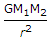M1 = mass of moon = 6.7 x 1022 kg

M2 = mass of earth = 6 x 1024 kg

r = distance between masses = 380 km

G = universal gravitational constant

= 6.7 x 10- 11 Nm2/Kg2

Let Q1 and Q2 be the charge on moon and earth respectively. Now the gravitational force must be balanced by the force of repulsion.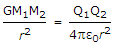Q1Q2 = 4pε0M1M2G

but Q2 = 12.5Q1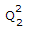= 12.5 x 4pω0M1M2G

Q2 = 6.11 x 1014

Q2 = 61.11 TC

Q1 = 4.89 TC.

5.
When x(n) is real then the Fourier series coefficients are correlated as __________ .
a-k = ak*
ak = ak*
ak+1 = ak*
a-k+1 = ak*